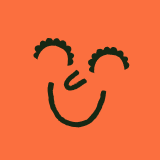teaching resource

# Mathematics Word Wall

A set of Mathematics related vocabulary to immerse students in mathematical terms.

Words include: circle, triangle, square, quadrilateral, pentagon, hexagon, heptagon, octagon, width, perimeter, area, length, volume, capacity, weight, sphere, cube, pyramid, grid, translation, reflection, rotation, angle, degree, factor, multiple, whole number, rounding, multiplication, division, addition, subtraction, remainder, fraction, decimal, number line, numerator, denominator, place value, less than, greater than, probability, data, observation, column graph, bar graph, table, line graph, pie chart, count forwards, count backwards, number before, number after, more than, less than, zero, ones, groups of ten, tens, is the same as, coins, notes

Would you like this resource with matching diagrams?

### teaching resource

#### Mathematics Word Wall with Diagrams

A set of 60 Mathematics-related vocabulary words with accompanying diagrams to explain key mathematical terms across three strands.22 pagesYears: 5 - 7

Write a review to help other teachers and parents like yourself. If you'd like to request a change to this resource, or report an error, select the corresponding tab above.

•·

These mathematics word wall cards would be great with the addition of a symbol to assist early readers eg - minus/takeaway + add/plus etc

•·

Hi Tricia, All of our Word Walls are editable and give you the option of adding additional cards if you need. Just hit the Customise button above and scroll to the bottom to add your own content. If there is anything else I can assist you with, please don't hesitate to contact me.

•·

Could you please have matching diagrams.